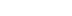/
article

# In-depth: Extracting dominant light from Spherical Harmonics

In this reprinted #altdevblogaday in-depth piece, game programmer Simon Yeung looks at extracting the dominant directional light from spherical harmonics-projected light, to fake specular lighting.
[In this reprinted #altdevblogaday in-depth piece, game programmer Simon Yeung looks at extracting the dominant directional light from spherical harmonics-projected light, to fake specular lighting.] Introduction Spherical Harmonics(SH) functions can represent low frequency data such as diffuse lighting, where those high frequency details are lost after projected to SH. Luckily we can extract a dominant directional light from SH coefficients to fake specular lighting. We can also extract more than 1 directional light from SH coefficients, but this post will only focus on extracting 1 dominant light, those interested can read Stupid Spherical Harmonics (SH) Tricks for the details. A webGL demo is provided at the last section which will only extract 1 directional light. Extracting dominant light direction We can get a single dominant light direction from the SH projected environment lighting, Le. Consider we approximate the environment light up to band 1 (i.e. l=1):Finding the dominant light direction is equivalent to choose an incoming direction, ω, so that Le(ω)is maximized. In other words, cosθ should equal 1:So we can extract the dominant light direction for a single color channel. Finally the dominant light direction can be calculated by scaling each dominant direction for RGB channels using the ration that converts color to grayscale:Extracting dominant light intensity After extracting the light direction, the remaining problem is to calculate the light intensity. That means we want to calculate an intensity s, so that the error between the extracted light and the light environment is at a minimum (Le is the original environment light while Ld is the directional light):To minimize the error, differentiate the equation and solve it to equal zero:If both lighting functions are projected into SH, the intensity can be simplified to:The next step is to project the directional light (with unit intensity) into SH basis (ci is the SH coefficient of the projected directional light):Therefore the SH coefficients of projected directional light can be calculated by substituting the light direction into the corresponding SH basis function. As the SH projected directional light is in unit intensity, we want to scale it with a factor so that the extracted light intensity s is the light color that can be ready for use in a direct lighting equation, which is defined as (detail explanation can be found in ):
For artist convenience, clight does not correspond to a direct radiometric measure of the light's intensity; it is specified as the color a white Lambertian surface would have when illuminated by the light from a direction parallel to the surface normal (lc = n).
So we need to calculate a scaling factor, c, that scales the SH projected directional light as such:We can project both L(ω) and (n . ω) into SH to calculate the integral. To project the transfer function (n . ω) into SH, we can first align the n to +Z-axis, which is zonal harmonics, then we can rotate the ZH coefficient into any direction using the equation:The ZH coefficients of (n . ω) are: (note that the result is different from Stupid Spherical Harmonics (SH) Tricks in the Normalization section as we have taken the π term outside the integral):Then rotate the ZH coefficients such that the normal direction equals the light direction, ld (because we need ld = n as stated above):Finally we can go back to compute the scaling factor, c, for the SH projected directional light (we calculate up to band=2):Therefore the steps to extract the dominant light intensity are first to project the directional light into SH with a scaling factor c, and then light color, s, which can be calculated by:WebGL Demo A webGL demo (you'll need a webGL enabled browser such as Chrome) is provided to illustrate how to extract a single directional light to fake the specular lighting from the SH coefficient. The specular lighting is calculated using the basic Blinn-Phong specular team for simplicity reasons. Other specular lighting equation can be used such as those physically plausible (The source code can be downloaded from here.).Screenshot of the demo
Conclusion Extracting the dominant directional light from SH projected light is easy to compute with the following steps: First, calculate the dominant light direction. Second, project the dominant light into SH with a normalization factor. Third, calculate the light color. The extracted light can be used for specular lighting to give an impression of high frequency lighting. References
 Stupid Spherical Harmonics (SH) Tricks: http://www.ppsloan.org/publications/StupidSH36.pdf
 PI or not to PI in game lighting equation: http://seblagarde.wordpress.com/2012/01/08/pi-or-not-to-pi-in-game-lighting-equation/  March of the Froblins: Simulation and Rendering Massive Crowds of Intelligent and Detailed Creatures on GPU: http://developer.amd.com/documentation/presentations/legacy/Chapter03-SBOT-March_of_The_Froblins.pdf  Pick dominant light from sh coeffs: http://sourceforge.net/mailarchive/message.php?msg_id=28778827
[This piece was reprinted from #AltDevBlogADay, a shared blog initiative started by @mike_acton devoted to giving game developers of all disciplines a place to motivate each other to write regularly about their personal game development passions.]

### Latest Jobs

#### Studio Pixanoh LLC

Los Angeles, California
05.20.22
Combat Designer

#### Treyarch

Playa Vista, California or Vancouver, BC
05.20.22
AI Engineer

Remote
05.20.22

#### Remedy Entertainment

Helsinki Metropolitan Area, Finland
05.23.22
Rigging Artist
More Jobs